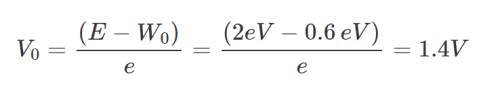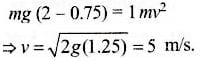JEE  >  Sample BITSAT Physics Test

# Sample BITSAT Physics Test - JEE

Test Description

## 40 Questions MCQ Test BITSAT Mock Tests Series & Past Year Papers - Sample BITSAT Physics Test

Sample BITSAT Physics Test for JEE 2023 is part of BITSAT Mock Tests Series & Past Year Papers preparation. The Sample BITSAT Physics Test questions and answers have been prepared according to the JEE exam syllabus.The Sample BITSAT Physics Test MCQs are made for JEE 2023 Exam. Find important definitions, questions, notes, meanings, examples, exercises, MCQs and online tests for Sample BITSAT Physics Test below.
Solutions of Sample BITSAT Physics Test questions in English are available as part of our BITSAT Mock Tests Series & Past Year Papers for JEE & Sample BITSAT Physics Test solutions in Hindi for BITSAT Mock Tests Series & Past Year Papers course. Download more important topics, notes, lectures and mock test series for JEE Exam by signing up for free. Attempt Sample BITSAT Physics Test | 40 questions in 50 minutes | Mock test for JEE preparation | Free important questions MCQ to study BITSAT Mock Tests Series & Past Year Papers for JEE Exam | Download free PDF with solutions
 1 Crore+ students have signed up on EduRev. Have you?
Sample BITSAT Physics Test - Question 1

### If E₀ represents the peak value of the voltage in an A.C. circuit, the r.m.s. value of the voltage will be

Detailed Solution for Sample BITSAT Physics Test - Question 1
For an a.c. circuit
Er.m.s=Eo/√2
Sample BITSAT Physics Test - Question 2

### After two hours, one sixteenth of the starting amount of a certain radioactive isotope remained undecayed. The half of the isotope is

Detailed Solution for Sample BITSAT Physics Test - Question 2
Use the equation of first order reaction lnCt=lnC0-kt. where Ct is the concentration at given time andC0 is the initial concentration and t is the given time here Ct=C0/16 and t=2 on solving you will get k =2ln2 and half life =ln2/k on solving you will get t=30 minutes
Sample BITSAT Physics Test - Question 3

### A freshly prepared radioactive sample of half-lif 1 hour emits radiations that are 128 times as intense as the permissible safe limit. The minimum time after which this sample can be safely used is

Sample BITSAT Physics Test - Question 4

In a Meter-bridge with a standard resistance of 5 Ω in the left gap, the ratio of balancing lengths on the Meter bridge wire is 2:3. The unknown resistance is

Sample BITSAT Physics Test - Question 5

A uniform resistance wire of length L and diameter d has a resistance R. Another wire of same material has length 4 L and diameter 2 d, the resistance will be

Sample BITSAT Physics Test - Question 6

What is the stopping potential when the metal with work function 0.6 eV is illuminated with the light of 2 eV?

Detailed Solution for Sample BITSAT Physics Test - Question 6where Vo is the Stopping potential.
Sample BITSAT Physics Test - Question 7

Which of the following is a correct statement?

Sample BITSAT Physics Test - Question 8

During a current change from 2 A to 4 A in 0.05 second, 8 V of emf is developed in a coil. the coefficient of self-induction is

Sample BITSAT Physics Test - Question 9

Five equal capacitors connected in series have a resultant capacitance of 4 μ F. The total energy stored in these, when these are connected in parallel and charged to 400V is

Sample BITSAT Physics Test - Question 10

A body of 2 gm carrying a charge of 0·1 μ C starts from rest at the positive plate and moves to the negative plate of a parallel plate condenser connected to a voltage supply of 30KV. The final velocity is

Sample BITSAT Physics Test - Question 11

A heavy uniform chain lies on a horizontal table-top. If the coefficient of friction between the chain and table surface is 0.25, then the maximum fraction of length of the chain, that can hang one edge of the table is

Sample BITSAT Physics Test - Question 12

Who among the following gave first the experimental value of G ?

Sample BITSAT Physics Test - Question 13

In a closed room, heat transfer takes place by

Detailed Solution for Sample BITSAT Physics Test - Question 13
Convection occurs when particles with a lot of heat energy in a liquid or gas move and take the place of particles with less heat energy. Heat energy is transferred from hot places to cooler places by convection. Liquids and gases expand when they are heated.
Sample BITSAT Physics Test - Question 14

In thermal equilibrium, the average velocity of gas molecules is

Sample BITSAT Physics Test - Question 15

If 10% of the current passes through a moving coil galvanometer of resistance 99 ohm, then the shunt resistance will be

Sample BITSAT Physics Test - Question 16

Ferromagnetic materials used in a transformer must have

Sample BITSAT Physics Test - Question 17

A liquid flows through a pipe of non-uniform cross-section. If A₁ and A₂ are the cross-sectional areas of the pipe at two points, the ratio of velocities of the liquid at these points will be

Sample BITSAT Physics Test - Question 18

In a liquid flowing through a pipe, the pressure difference between its ends is made half and radius is doubled. If the initial rate of flow of liquid was Q, then new rate of flow of liquid through the pipe is

Sample BITSAT Physics Test - Question 19

How much force is required to produce an elongation of 0.2% in the length of a brass wire of diameter 0.6 mm? (Take Young's modulus for brass = 0.9*1011 N-m⁻2)

Sample BITSAT Physics Test - Question 20

A ball is dropped from a bridge at a height of 176.4 m over a river. After 2 s, a second ball is thrown straight downwards. What should be the initial velocity of the second ball so that both hit the water simultaneously ?

Sample BITSAT Physics Test - Question 21

A car is moving at a speed of 72 km−h⁻1. The diameter of its wheels is 0.5 m. If the wheels are stopped in 20 rotations by applying brakes, then the angular retardation produced by the brakes is

Sample BITSAT Physics Test - Question 22

Two bodies are projected with the same velocity. If one is projected at an angle of 30o and the other at an angle of 60o to the horizontal, the ratio of the maximum heights reached is

Sample BITSAT Physics Test - Question 23

A body of mass 2 kg is moving with a velocity of 8m/s on a smooth surface.if it is to be brought to rest in 4 seconds,then the force to be applied is?

Sample BITSAT Physics Test - Question 24

The ability of eye to focus near as well as distant objects is called

Sample BITSAT Physics Test - Question 25

A child is sitting on a swing. Its minimum and maximum heights from the ground are 0.75 m and 2m respectively. Its maximum speed will be

Detailed Solution for Sample BITSAT Physics Test - Question 25

Drop in P.E. =  maximum K.E.Sample BITSAT Physics Test - Question 26

When a force of 0.1 N is applied, the spring is stretched by 1.5 cm. The spring is cut into three parts and one part is stretched by 3 cm. Find the force required for doing so.

Sample BITSAT Physics Test - Question 27

A mass is revolving in a circle which is in the plane of paper. The direction of angular acceleration is

Sample BITSAT Physics Test - Question 28

An air bubble is contained inside water. It behaves as a

Sample BITSAT Physics Test - Question 29

When a sphere of moment of inertia I about an axis through its centre of gravity and mass m rolls down from rest, on an inclined plane without slipping, its kinetic energy is

Sample BITSAT Physics Test - Question 30

A cylinder of mass 500 g and radius 10 cm has moment of inertia about its central axis in kg-m2 is equal to

Sample BITSAT Physics Test - Question 31

A battery of e.m.f. 10 V and internal resistance 0.5 ohm is connected across a variable resistance R. The value of R for which the power delivered in it is maximum is given by

Sample BITSAT Physics Test - Question 32

An ideal monoatomic gas at 27oC is compressed adiabatically to 8/27 times of its original volume. The increase in temperature of the gas is

Sample BITSAT Physics Test - Question 33

In a thermodynamic system, ∆u represents an increase in its internal energy and ∆W work done by the system. Which of the following statements is true?

Sample BITSAT Physics Test - Question 34

A suitable unit for gravitational constant is

Sample BITSAT Physics Test - Question 35

If the ratio of intensities of two waves causing interference be 9:4, then the ratio of maximum and minimum intensities will be

Sample BITSAT Physics Test - Question 36

Laser light is considered to be coherent as it consists of

Sample BITSAT Physics Test - Question 37

A tuning fork whose frequency as given by manufacturer is 512 Hz is being tested with an accurate oscillator. It is found that the fork produces a beat of 2 Hz when oscillator reads 514 Hz but produces a beat of 6 Hz when oscillator reads 510 Hz. The actual frequency of fork is

Sample BITSAT Physics Test - Question 38

The tension in a piano wire is 10N. What should be the tension in the wire to produce a note of double the frequency

Sample BITSAT Physics Test - Question 39

A block of mass 10 kg is moving in x-direction with a constant velocity of 10 m−s⁻1. It is subjected to a retarding force F = 0.1x J-m⁻1 during its travel from x = 20 m to x = 30 m. Its final kinetic energy will be

Sample BITSAT Physics Test - Question 40

What remains constant in the field of central force

## BITSAT Mock Tests Series & Past Year Papers

2 videos|14 docs|143 tests
Information about Sample BITSAT Physics Test Page
In this test you can find the Exam questions for Sample BITSAT Physics Test solved & explained in the simplest way possible. Besides giving Questions and answers for Sample BITSAT Physics Test, EduRev gives you an ample number of Online tests for practice

## BITSAT Mock Tests Series & Past Year Papers

2 videos|14 docs|143 tests(Scan QR code)